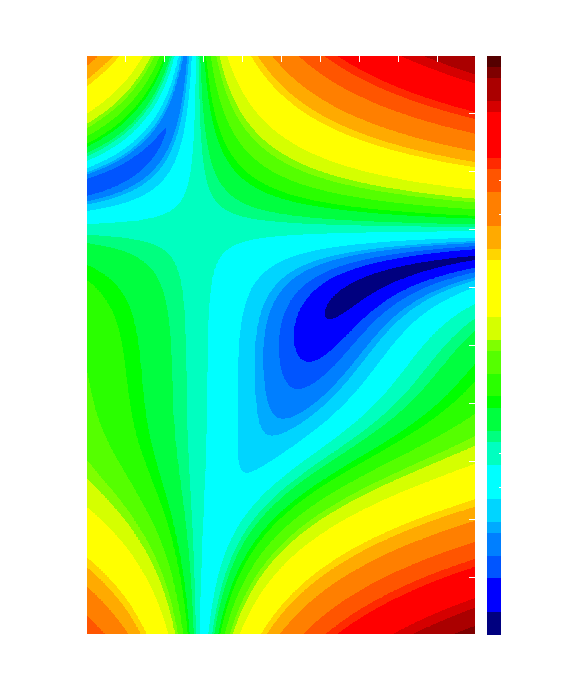## The Unconstrained NLO Problem

The Problem: min f(x), n=dim(x)The picture shows the level set representation of Beale's testfunction

f(x,y)=(1.5-x(1-y))^2+(2.25-x(1-y^2))^2+(2.625-x(1-y^3))^2.
It has a unique global minimizer at (3,0.5) and a saddle point at (0,1). There is a further saddle point at (0.100538,-2.644514). For x=0 or y=1 it is constant with value 14.203125. In x<0 there exists an arc y(x)>0, where f decreases monotonically as x-> -INF. Initial values near x=0 for large |y| or x<0, y>0 will let descent methods fail.

The methods listed here all restrict themselves to finding one solution of grad f(x)=0. For trust region based methods one often can show that this solution automatically satisfies the second order necessary conditions.

f is a "general" twice continuously differentiable function, but gradient is not available:

The methods implicitly all assume that f is twice continuously differentiable. They may fail or converge very slowly if this is not the case. Read M. Powell's and M. Wright's papers on Direct Search methods as well as M. Powell's paper on optimization without derivatives.

 VRBBO Stochastic Matlab package with claims to near global optimization FMIN Fortran version of Brent's localmin (f77) PRIMA Updated implementation of MJD Powell's DFO codes in several languages UNCMND quasi-Newton method by Schnabel et al (f77) C++ version WEDGE Interpolation/trust region method for moderate dimensions(Matlab) netlib/opt/praxis f depends on few variables, the "principal axis method", tries to approximate the curvature of f MCToolbox three direct search methods incl Nelder-Mead, Matlab nelmead f depends on few variables, Nelder-Mead simplex-search method (no sound theoretical basis), f77 netlib/opt/subplex f depends on few variables, modification of the Nelder-Mead simplex-search method (no sound theoretical basis), (Matlab version) fminsi modified Nelder-Mead, LGPL-licensed, source, testdriver DFO derivative free method of Conn, Scheinberg, and Toint HOPSPack General derivative-free framework for unconstrained and linearly constrained problems, parallel, GPL, C++

f is only Lipschitz continuous or less: n not too large

 BFO A trainable derivative-free solver for mixed-integer bound-constrained problems (Matlab) GradSamp Nonsmooth, nonconvex optimization by gradient sampling, by M. Overton et al; needs one of three QP solvers (Matlab) LMBM Limited Memory Bundle Method, f77, Matlab interface, testproblems, bound-constrained version GRANSO for nonsmooth, nonconvex optimization subject to nonsmooth, nonconvex constraints, based on a BFGS-SQP method (Matlab) SolvOpt Solves nonsmooth unconstrained and constrained problems of moderate dimensions (python). OBOE Oracle-Based Optimization Engine for convex problems, uses Proximal-ACCPM interior point method, C++ PBUN/PNEW Different f77 routines for Lipschitz continuous unconstrained and linearly constrained problems NSO various software for large-scale nonsmooth optimization (Fortran) VXQR1 Derivative-free unconstrained optimization based on QR factorizations (Matlab)

The gradient of f is available or finite difference approximations are applicable :

n not too large (= some hundred say)

 netlib/toms/500 the Shanno-Phua version of BFGS plus CG netlib/toms/611 trust region BFGS, this is a reliable and fairly efficient code. netlib/toms/739 tensor model quasi-Newton method, may be better for strongly nonquadratic f NMTR trust-region Newton, requires gradient UCMINF several methods, paper (Matlab) CG_DESCENT A new conjugate gradient method with guaranteed descent (f77/C) csminwel BFGS with some robustness against nonsmoothness (Matlab)

f is convex differentiable, n large:

 netlib/opt/ve08 f is a sum of convex differentiable functions each of which has considerably less variables than n (partially separable problem) (also for bound constraints), special quasi Newton method

n large, f general, but not too wildly behaved :

 netlib/toms/630 updated version in f90 A combined limited memory qN/cg method (degrades for nonconvex f, nevertheless applicable; for ill-conditioned problem an individual preconditioner must be provided) MINPACK-2 A limited memory quasi Newton method. Directories contain software, drivers and manuals. (dcsrch.f, the step-size-algorithm, must be added; to be found e.g. in the following code) LBFGS Java f90 a limited memory quasi Newton method using a new matrix representation; directory contains software, drivers and a user's manual. Not to be recommended for ill-conditioned problems. Add your own preconditioner! LBFGS-B Matlab wrapper needs C++ and Fortran compiler CG+ three basic cg methods, POBLANO Matlab toolbox PREQN preconditioning package LM Several limited memory f77-routines for unconstrained and box-constrained optimization; also for least squares

n large, f strongly nonquadratic :

 netlib/opt/tn C-version Nash's truncated Newton method based on the Lanczos method, without explicit eigenvalue computation. takes directions of negative curvature into account. netlib/toms/702 truncated Newton based on the Lanczos process TRON trust region Newton, preconditioned cg, also for bound constraints

f noisy :

 CMA-ES Evolution Strategy with Covariance Matrix Adaptation (Matlab) SNOBFIT Stable Noisy Optimization by Branch and FIT (Matlab) IMFIL implicit filtering subject to box constraints and with multiple minima (Matlab) DIRECT the DIRECT algorithm (in Matlab) VTDIRECT95 Serial and Parallel Codes for the Global Optimization Algorithm DIRECT (Fortran 95) netlib/opt/praxis often applied to noisy problems although not directly designed for such PRIMA Updated implementation of MJD Powell's DFO codes in several languages netlib/opt/subplex f depends on few variables, modification of the Nelder-Mead simplex-search method (no sound theoretical basis), (Matlab version)

f expensive to evaluate :

 RBFOpt radial basis function library for black box (derivative free) optimization of functions with costly function evaluation (Python) Scatter Search all the C code from this book SPACE global optimization through meta-modeling: fitting, cross-validation, prediction, minimization etc (C++) Spacemap Optimization using Surrogate Models (Matlab) STK Matlab/Octave toolbox to construct a kriging approximation (surrogate) to computer models

f complex :

 Complex Optimization Toolbox MATLAB toolbox for optimization of complex variables